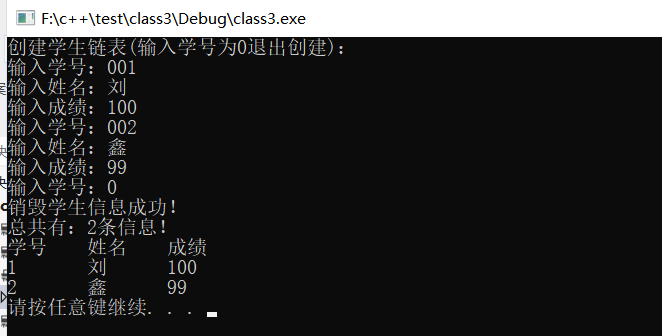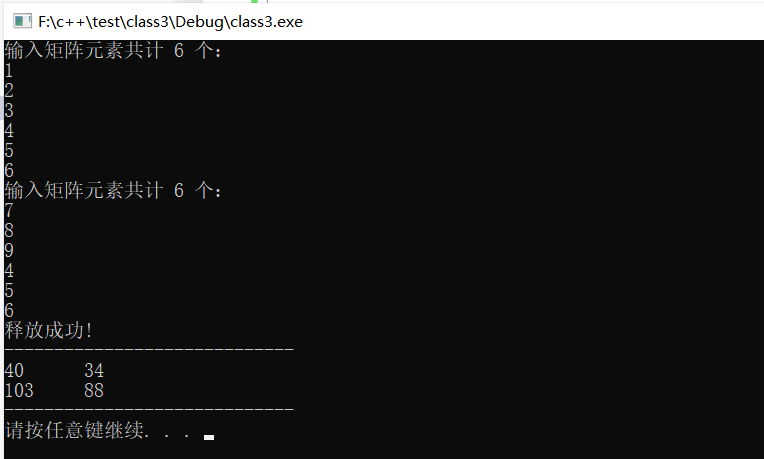## C++第十四次作业（静态成员-static_友元-friend_运算符重载-operator_内联函数-inline_this 指针_指向类的指针 -＞）_刘鑫磊up的博客-程序员宅基地

习题1：c++学生链表的创建与输出，定义学生类，学生链表类，后者作为前者的友类

## 学习一下

### 一：c++静态成员（static）

``````1.为什么使用静态成员来实现同一类的对象之间的数据共享
（1）全局变量：安全隐患、违背OOP“数据隐藏”的原则
（2）一般的数据成员：有数据冗余，浪费空间
（3）静态成员：本类的所有对象共同拥有一个存储“总数”的数据成员
（4）如果不存在其他的初始化语句，在创建第一个对象时，所有的静态数据都会被初始化为零
（5）我们不能把静态成员的初始化放置在类的定义中
但是可以在类的外部通过使用范围解析运算符 :: 来重新声明静态变量从而对它进行初始化
参考：https://www.runoob.com/cplusplus/cpp-static-members.html

2.静态数据成员的特点
(1）静态数据成员为本类所有对象共有，是“类属性”而非“实例属性”
不专属于任一对象，为所有对象共享
（2）静态数据成员单独存储并只存储一份
（3）静态数据成员的初始化在类定义体外
（4）静态成员函数只能直接访问静态成员数据（或函数），否则应通过参数传递对象
static void ShowINF(Student &P )
{	cout<<P.ID<<’\t’<<P.Name<<’\t’<<P.Cscore<<endl;	}
（5）静态成员函数没有this指针，而非静态成员函数总是某具体对象调用
（6）在没有创建任何对象时，可以使用静态成员函数输出静态数据成员的值
（7）当我们声明类的成员为静态时，这意味着无论创建多少个类的对象，静态成员都只有一个副本
(8)静态成员变量在类中仅仅是声明，没有定义，所以要在类的外面定义，实际上是给静态成员变量分配内存
如果不加定义就会报错，初始化是赋一个初始值，而定义是分配内存

3.静态数据成员的使用，静态成员的初始化
class Student
{
private:
unsigned ID;
string Name;
double CScore;
static unsigned SCounts;    //静态数据
public:
Student(unsigned cID=0,string cName="",double ccscore=0);
Student(Student &s);
void ShowINF();
unsigned Counts(){return SCounts;}    //调用
~Student();
};
//实现部分
//………………………………

int Box::objectCount = 0;

4.静态成员函数
4.1 如果把函数成员声明为静态的，就可以把函数与类的任何特定对象独立开来
4.2 静态成员函数即使在类对象不存在的情况下也能被调用
静态函数只要使用类名加范围解析运算符 :: 就可以访问
4.3 静态成员函数只能访问静态成员数据、其他静态成员函数和类外部的其他函数
只能访问静态成员（包括静态成员变量和静态成员函数）
4.4 静态成员函数有一个类范围，他们不能访问类的 this 指针

5.类中特殊成员变量的初始化问题
5.1 常量变量：必须通过构造函数参数列表进行初始化
5.2 引用变量：必须通过构造函数参数列表进行初始化
5.3 普通静态变量：要在类外通过"::"初始化
5.4 静态整型常量：可以直接在定义的时候初始化
5.5 静态非整型常量：不能直接在定义的时候初始化。要在类外通过"::"初始化``````

### 二：c++友元、友元成员和友元类（friend）

``````1.友元特点
（1）因为类外只能直接访问public变量，如果要保证面向对象的思想且必须遵守OOP数据封装隐藏的含义
若要把所有数据定义为共有变量，在类外也可以访问类的私有变量，则可以利用——友元
（2）友元函数是一个普通函数，不是类的成员函数
（3）在public和private部分声明无区别
（4）友元函数无this指针
(5)类的友元函数是定义在类外部
但有权访问类的所有私有（private）成员和保护（protected）成员
(6)尽管友元函数的原型有在类的定义中出现过，但是友元函数并不是成员函数

2.友元的基本语法
class Point
{
private :
double x,y;
public:
Point(double cx=0.0,double cy=0.0):x(cx),y(cy){ }
Point(Point &p);
void Show();
void Getxy(double &dx,double &dy)//不方便
{	dx=x ;	dy=y;	}
~Point(){ }

friend double DisTance(Point A,Point B); //友元函数
friend Point MidPoint(Point A,Point B); //友元函数
};
//成员函数实现
//其它函数的实现

3.友元成员：一个类的成员函数可作为另一个类的友元

4.友元类：友元也可以是一个类，该类被称为友元类
一个类可以成为另一个类的友元，在这种情况下，整个类及其所有成员都是友元

5.友元函数：友元可以是一个函数，该函数被称为友元函数``````

### 三：c++运算符的重载（operator）

``````1. 重载运算符和重载函数的定义
1.1 C++ 允许在同一作用域中的某个函数和运算符指定多个定义，分别称为函数重载和运算符重载
1.2 重载声明是指一个与之前已经在该作用域内声明过的函数或方法具有相同名称的声明
但是它们的参数列表和定义（实现）不相同

2.函数重载：在同一个作用域内，可以声明几个功能类似的同名函数，但是这些同名函数的形式参数（指参数的个数、类型或者顺序）必须不同。您不能仅通过返回类型的不同来重载函数
class printData
{
public:
void print(int i)
{
cout << "整数为: " << i << endl;
}

void print(double  f)
{
cout << "浮点数为: " << f << endl;
}

void print(char c[])
{
cout << "字符串为: " << c << endl;
}
};

int main(void)
{
printData pd;

// 输出整数
pd.print(5);
// 输出浮点数
pd.print(500.263);
// 输出字符串
char c[] = "Hello C++";
pd.print(c);

return 0;
}

3.运算符重载
3.1 您可以重定义或重载大部分 C++ 内置的运算符。这样，您就能使用自定义类型的运算符
3.2 重载的运算符是带有特殊名称的函数，函数名是由关键字 operator 和其后要重载的运算符符号构成的
Box operator+(const Box&);
3.3 与其他函数一样，重载运算符有一个返回类型和一个参数列表
3.4 大多数的重载运算符可被定义为普通的非成员函数或者被定义为类成员函数
类的非成员函数：Box operator+(const Box&, const Box&);

// 重载 + 运算符，用于把两个 Box 对象相加
Box operator+(const Box& b)
{
Box box;
box.length = this->length + b.length;
box.height = this->height + b.height;
return box;
}

4.运算符重载规则：
您可以重定义或重载大部分 C++ 内置的运算符。这样，您就能使用自定义类型的运算符
4.1 被重载的运算符必须是已经存在的C++运算符，不能重载自己创建的运算符；
4.2 运算符被重载之后，原有功能仍然保留。只是扩展了原有功能；
4.3 重载不能改变运算符运算对象的个数。
+运算符具有两个操作数，在+运算符函数作为CTime的成员函数的时候
有一个参数是隐含的，也就是当前的对象，使用this来引用
另一个参数通过函数参数指定。

5.关于运算符重载
5.1 可以重载的运算符
1）算术运算符：+，-，*，/，%，
2）逻辑运算符：&&，||，！
3）关系运算符：>，<，=，>=，<=，==，!=
4）位操作符：～，<<，>>，&，^），|
5）自增自减运算符：++，--
自增和自减都有前后之分，所以++和—都有两种重载方式。
6）复合赋值运算符：+=，-=，*=，/=，%=
7）其他：&、*、 () 、-> 、[]、.new/delete、>>、<<
5.2 不能重载的运算符
?: . * :: sizeof(这也是一个运算符)
5.3 不需要重载的运算符
=（赋值）和&(取地址符)

6.成员函数方式要求左侧的参数要与类类型相同。而普通函数则要求实参顺序与形参类型顺序一致
3.1 有的运算符必须定义为类的成员函数：=、[]、()
3.2 有的运算符不能定义为类的成员函数，只能定义为类的友元:<<、>>``````

1 一元运算符重载
2 二元运算符重载
3 关系运算符重载
4 输入/输出运算符重载
5 ++ 和 -- 运算符重载
6 赋值运算符重载
7 函数调用运算符 () 重载
8 下标运算符 [] 重载
9 类成员访问运算符 -> 重载

### 补充2：下面是不可重载的运算符列表

``````.：成员访问运算符
.*, ->*：成员指针访问运算符
::：域运算符
sizeof：长度运算符
?:：条件运算符
#： 预处理符号``````

### 补充3：下面是可重载的运算符列表

 双目算术运算符 + (加)，-(减)，*(乘)，/(除)，% (取模) 关系运算符 ==(等于)，!= (不等于)，< (小于)，> (大于>，<=(小于等于)，>=(大于等于) 逻辑运算符 ||(逻辑或)，&&(逻辑与)，!(逻辑非) 单目运算符 + (正)，-(负)，*(指针)，&(取地址) 自增自减运算符 ++(自增)，--(自减) 位运算符 | (按位或)，& (按位与)，~(按位取反)，^(按位异或),，<< (左移)，>>(右移) 赋值运算符 =, +=, -=, *=, /= , % = , &=, |=, ^=, <<=, >>= 空间申请与释放 new, delete, new[ ] , delete[] 其他运算符 ()(函数调用)，->(成员访问)，,(逗号)，[](下标)

### 四：内联函数（ inline）

``````1.内联函数是通常与类一起使用

2.如果一个函数是内联的，那么在编译时，编译器会把该函数的代码副本放置在每个调用该函数的地方

3.对内联函数进行任何修改，都需要重新编译函数的所有客户端
因为编译器需要重新更换一次所有的代码，否则将会继续使用旧的函

4.如果想把一个函数定义为内联函数，则需要在函数名前面放置关键字 inline

5.在调用函数之前需要对函数进行定义，内联函数的定义必须出现在内联函数第一次调用之前

6.如果已定义的函数多于一行，编译器会忽略 inline 限定符

7.在类定义中的定义的函数都是内联函数，即使没有使用 inline 说明符

8.引入内联函数的目的是为了解决程序中函数调用的效率问题
8.1程序在编译器编译的时候，编译器将程序中出现的内联函数的调用表达式用内联函数的函数体进行替换
8.2而对于其他的函数，都是在运行时候才被替代
8.3这其实就是个空间代价换时间的节省
8.4当函数被声明为内联函数之后, 编译器会将其内联展开, 而不是按通常的函数调用机制进行调用

9.在内联函数内不允许使用循环语句和开关语句

10.优缺点
优点：
当函数体比较小的时候, 内联该函数可以令目标代码更加高效.
对于存取函数以及其它函数体比较短, 性能关键的函数, 鼓励使用内联
缺点：
滥用内联将导致程序变慢. 内联可能使目标代码量或增或减, 这取决于内联函数的大小

11.谨慎对待析构函数, 析构函数往往比其表面看起来要更长, 因为有隐含的成员和基类析构函数被调用

12.有些函数即使声明为内联的也不一定会被编译器内联, 这点很重要;
比如虚函数和递归函数就不会被正常内联``````

### 五：this 指针

``````1.每一个对象都能通过 this 指针来访问自己的地址

2.this 指针是所有成员函数的隐含参数

3.因此，在成员函数内部，它可以用来指向调用对象

4.友元函数没有this指针，因为友元不是类的成员
只有成员函数才有 this 指针

5.当我们调用成员函数时，实际上是替某个对象调用它

6.当我们调用一个成员函数时，用请求该函数的对象地址初始化 this``````

### 六：指向类的指针（ ->）

``````1.访问指向类的指针的成员，需要使用成员访问运算符 ->，就像访问指向结构的指针一样

2.和指针一样，必须在使用指针之前，对指针进行初始化
// 保存第一个对象的地址
ptrBox = &Box1;

// 现在尝试使用成员访问运算符来访问成员
cout << "Volume of Box1: " << ptrBox->Volume() << endl;``````

## 习题1：c++学生链表的创建与输出，定义学生类，学生链表类，后者作为前者的友类

### 代码实现

``````#include <iostream>
#include <string>
using namespace std;

//先定义学生类，再定义学生链表类

//定义学生类
class Student
{
private:
unsigned ID;//学号
string Name;//姓名
double CScore;//成绩
static unsigned SCounts;//静态全局共享，记录几条数据
Student *next;//指针域
public:
Student(unsigned cID=0,string cName="",double ccscore=0);//学生类成员函数的实现
Student(Student &s);//拷贝
void SetValue();//输入
void ShowINF();//输出
static unsigned Counts(){return SCounts;}//计数
~Student();//析构
friend class StuList;//友元
};
//静态成员的初始化
unsigned Student::SCounts=0;

//学生类成员函数的实现
Student::Student(unsigned cID,string cName,double ccscore)
{
ID=cID;
Name=cName;
CScore=ccscore;
SCounts++;
}
//输入初始化
void Student::SetValue()
{
cout<<"输入学号：";cin>>ID;
cout<<"输入姓名：";fflush(stdin);getline(cin,Name);
cout<<"输入成绩：";cin>>CScore;
}
//拷贝构造函数
Student::Student(Student &s)
{
ID=s.ID;Name=s.Name;CScore=s.CScore;next=s.next;
}
//输出函数
void Student::ShowINF()
{
cout<<"学号："<<ID<<"\t姓名："<<Name<<"\t成绩："<<CScore<<endl;
}
//析构函数
Student::~Student()
{
cout<<"销毁学生信息成功！"<<endl;
SCounts--;
}
//-----------------------------------------------------------------------------
//学生链表类的定义
class StuList
{
private:
unsigned NodeCount;//计数
public:
StuList();//构造函数
void ShowList();//输出函数
~StuList();//析构函数
friend class Student;//友元
};

//构造函数
StuList::StuList()
{
Student *pnew=NULL;
NodeCount=0;
cout<<"创建学生链表(输入学号为0退出创建)："<<endl;
for(;;)
{
pnew=new Student;
pnew->next=NULL;
cout<<"输入学号：";
cin>>pnew->ID;
if(pnew->ID==0)
{
delete pnew;
break;
}
fflush(stdin);//清除键盘缓存区
cout<<"输入姓名：";
getline(cin,pnew->Name); fflush(stdin);
cout<<"输入成绩：";
cin>>pnew->CScore;
{
}else
{
//在头结点前面
{
}
//在尾结点后面
else if(pnew->ID>=End->ID)//当插入的结点>=最后一个
{
End->next=pnew;//那么就直接放在后面
End=pnew;
}
//否则就在中间
else{
while(pe)//当不到结尾 0
{
if(ps->ID<pnew->ID && pnew->ID<pe->ID)//这里说明在中间
{
pnew->next=pe;//中间的后面是pe
ps->next=pnew;//中间的前面是ps
}
ps=pe;//赋值
pe=ps->next;//依次取
}
}
}
NodeCount++;//计数
}
}

//输出链表
void StuList::ShowList()
{
if(pstu==NULL)
{
cout<<"链表为空！"<<endl;
return;
}
cout<<"总共有："<<NodeCount<<"条信息！"<<endl;
cout<<"学号\t姓名\t成绩"<<endl;
while(pstu)
{
cout<<pstu->ID<<"\t"<<pstu->Name<<"\t"<<pstu->CScore<<endl;
pstu=pstu->next;//依次取
}
}

//析构函数
StuList::~StuList()
{
{
cout<<"链表为空！"<<endl;
return;
}
while(paid)//对象 头结点不为空
{
delete paid;
}
cout<<"学生链表销毁！"<<endl;
}
int main()
{
StuList list;
list.ShowList();
system("pause");
return 0;
}``````

### 运行结果## 习题2：c++重载运算符‘*’实现两个矩阵的乘积;重载‘=’实现矩阵赋值

C++重载运算符实现矩阵的运算：https://blog.csdn.net/weixin_46108395/article/details/105810795

C++重载赋值操作符：http://www.weixueyuan.net/view/6383.html

### 代码实现

``````#include<iostream>
using namespace std;

class Matrix
{
public:
Matrix(unsigned cr=0,unsigned cc=0);//构造函数
void SetMatrixValue();//赋值
~Matrix();//析构函数
void ShowMatrix();//输出
Matrix operator *(Matrix &M);//重载*
void operator=(Matrix &M);//重载=

friend ostream &operator<<(ostream &output,const Matrix &M)//友元，重载<<，输出 ostream output
{
cout<<"-----------------------------"<<endl;
unsigned int i,j;
for(i=0;i<M.r;i++)//行
{
for(j=0;j<M.c;j++)//列
output<<M.a[i*M.c+j]<<'\t';//输出
output<<endl;
}
cout<<"-----------------------------"<<endl;
return output;
}
friend istream &operator>>(istream &input,Matrix &M)//友元，重载>>，输入iostream intput
{
unsigned int i,j;
cout<<"输入矩阵元素共计 "<<M.r*M.c<<" 个："<<endl;
for(i=0;i<M.r;i++)//行
{
for(j=0;j<M.c;j++)//列
input>>M.a[i*M.c+j];//输入
}
return input;
}
private:
double *a;
unsigned r,c;
bool IsEmpty;
};

//构造
Matrix::Matrix (unsigned cr,unsigned cc)
{
r=cr;c=cc;a=NULL;
if(r*c==0)//如果行列为0
{
IsEmpty=1;
return;//为空直接返回
}
a=new double[r*c];//那么申请一个空间
IsEmpty=0;
}

//输入矩阵的元素个数
void Matrix::SetMatrixValue ()
{
if(IsEmpty)//如果为空那么直接返回
return;
unsigned i,j;
cout<<"输入矩阵元素共计 "<<r*c<<" 个："<<endl;
for(i=0;i<r;i++)//行
{
for(j=0;j<c;j++)//列
cin>>a[i*c+j];
}
}

//析构函数
Matrix::~Matrix()
{
cout<<"释放成功!"<<endl;
if(IsEmpty==1)
{
cout<<"矩阵为空！"<<endl;
return;
}
delete []a;
}

//输出打印矩阵
void Matrix::ShowMatrix ()
{
if(IsEmpty==1)
{
cout<<"矩阵为空!"<<endl;
return;
}
unsigned i,j;
for(i=0;i<r;i++)//行
{
for(j=0;j<c;j++)//列
cout<<a[i*c+j]<<'\t';
cout<<endl;
}
}

//重载*
Matrix Matrix::operator*(Matrix &M)
{
//M K   K N
if((this->c!=M.r )||(this->IsEmpty)||(M.IsEmpty))
{
cout<<"无法实现矩阵乘积"<<endl;system("pause");return Matrix(0,0);
}
Matrix *C=new Matrix(this->r,M.c);//开辟新的空间 r c
unsigned i,j,k;
for(i=0;i<r;i++)//第一个矩阵的行——i
{
for(j=0;j<M.c;j++)//第二个矩阵的列——j
{
C->a[i*M.c+j]=0;
for(k=0;k<c;k++)//公共的：第一个矩阵的列或者第二个矩阵的行——k
C->a[i*M.c+j]+=a[i*c+k]*M.a[k*M.c+j];//i*j=第一个i*j  * 第二个i*j
}
}
return *C;
}

//重载=
void Matrix::operator=(Matrix &M)
{
r=M.r;c=M.c;
IsEmpty=M.IsEmpty ;
if(IsEmpty)//如果为空直接返回
return;
a=new double[r*c];//开辟新的地址
unsigned i,j;
for(i=0;i<r;i++)//行
for(j=0;j<c;j++)//列
a[i*c+j]=M.a[i*c+j];//这里相当于直接赋值
}

int main()
{
Matrix A(2,3),B(3,2),C;
//A.SetMatrixValue ();B.SetMatrixValue ();
cin>>A>>B;
//重载=和*
C=A*B;//A*B本质上是A.operator*(B);
//C.operator=(A*B);
//C.ShowMatrix ();
cout<<C;
system("pause");
return 0;
}
``````

### 运行结果### C语言-编写程序，根据输入的学生成绩给出相应的等级，大于或等于90分以上的等级为A，60分以下的等级为E，其余每10分为一个等级。（switch语句）_c语言根据输入的学生成绩给出相应等级-程序员宅基地

#include<stdio.h>main(){ int g; printf("Enter a mark:"); scanf("%d",&g);//g中存放学生的成绩 printf("g=%d:",g); switch(g) { case 10: case 9:printf("A\n");break; case 8:printf("B\n");break;..._c语言根据输入的学生成绩给出相应等级

### LVDS 低电压差分信号_为什么lvds要差分电阻-程序员宅基地

LVDS：Low Voltage Differential Signaling，低电压差分信号。　　LVDS传输支持速率一般在155Mbps（大约为77MHZ）以上。　　LVDS是一种低摆幅的差分信号技术，它使得信号能在差分PCB线对或平衡电缆上以几百Mbps的速率传输，其低压幅和低电流驱动输出实现了低噪声和低功耗。　　IEEE在两个标准中对LVDS信号进行了定义。ANSI/TIA/E_为什么lvds要差分电阻

### http错误-程序员宅基地

400 Bad request（错误请求）　　401.1 Logon failed（登录失败）　　401.2 Logon failed due to server configuration（由于服务器配置，登录失败）　　401.3 Unauthorized due to ACL on resource（由于资源上的 ACL，未授权）　　401.4...

### pip升级后不能查看版本，不能安装包-程序员宅基地

1.问题：把pip2通过 sudo pip2 install --upgrade pip升级为18.1后，查看版本pip2 -V，显示Traceback (most recent call last): File "/usr/bin/pip2", line 9, in &lt;module&gt; from pip import mainImportError: cannot...

### 【安卓学习之第三方库】 android安卓最常用的Jar包收集_aspectjrt-1.7.3.jar-程序员宅基地

█ 【安卓学习之第三方库】 android安卓最常用的Jar包收集█ 相关文章：-　　●　【安卓学习之第三方库】库的使用2-jar类库的使用(以dom4j为例)和升级(以极光推送为例)█ 读前说明：-　　●　本文通过学习别人写demo,学习一些课件,参考一些博客,’学习相关知识，如果涉及侵权请告知　●　本文只简单罗列相关的代码实现过程　●　涉及到的逻辑以及说明也只是简单介绍，主要当做笔记，了解过程而已█ 我的问题：-　　●　现在如果继续使用eclipse （ADT）开发andr_aspectjrt-1.7.3.jar

### table布局-程序员宅基地

开辟了博客园,观察样式有感,当用鼠标缩小浏览器大小时,可以看到主内容区内右边的区域是固定的,左边的自适应,这里用到了table.　　　　大概是这样　　.content{　　　　width:100%;　　　　display:table;　　}　　.leftBox{　　　　display:table-cell;　　}　　.rightBox{　　..._table写布局